Chapter 2.4, Problem 31E### Elementary Geometry for College St...

6th Edition
Daniel C. Alexander + 1 other
ISBN: 9781285195698

#### Solutions

Chapter
Section### Elementary Geometry for College St...

6th Edition
Daniel C. Alexander + 1 other
ISBN: 9781285195698
Textbook Problem
1 views

# Given: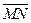and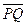intersect Prove: at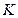;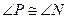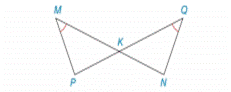To determine

To prove:

PN

Explanation

Given:

MN¯ and PQ¯ intersect at k and MQ.

Figure (1)

Approach:

(1) The vertical angles formed by the intersection of two lines are congruent.

(2) If two angles of a triangle are congruent to the two angles of another triangle, the third angle is also congruent to each other.

Calculation:

The MKP and QKN are formed by intersection of lines MN¯ and PQ¯

### Still sussing out bartleby?

Check out a sample textbook solution.

See a sample solution

#### The Solution to Your Study Problems

Bartleby provides explanations to thousands of textbook problems written by our experts, many with advanced degrees!

Get Started

#### Calculate y'. 42. y=(x+)4x4+4

Single Variable Calculus: Early Transcendentals, Volume I

#### 22. If the cost function for a commodity is graph the marginal cost function.

Mathematical Applications for the Management, Life, and Social Sciences

#### The graph of x = cos t, y = sin2 t is:

Study Guide for Stewart's Single Variable Calculus: Early Transcendentals, 8th